Searching Algorithms

# Searching Algorithms

Test Description

## 10 Questions MCQ Test GATE Computer Science Engineering(CSE) 2023 Mock Test Series | Searching Algorithms

Searching Algorithms for Computer Science Engineering (CSE) 2023 is part of GATE Computer Science Engineering(CSE) 2023 Mock Test Series preparation. The Searching Algorithms questions and answers have been prepared according to the Computer Science Engineering (CSE) exam syllabus.The Searching Algorithms MCQs are made for Computer Science Engineering (CSE) 2023 Exam. Find important definitions, questions, notes, meanings, examples, exercises, MCQs and online tests for Searching Algorithms below.
Solutions of Searching Algorithms questions in English are available as part of our GATE Computer Science Engineering(CSE) 2023 Mock Test Series for Computer Science Engineering (CSE) & Searching Algorithms solutions in Hindi for GATE Computer Science Engineering(CSE) 2023 Mock Test Series course. Download more important topics, notes, lectures and mock test series for Computer Science Engineering (CSE) Exam by signing up for free. Attempt Searching Algorithms | 10 questions in 30 minutes | Mock test for Computer Science Engineering (CSE) preparation | Free important questions MCQ to study GATE Computer Science Engineering(CSE) 2023 Mock Test Series for Computer Science Engineering (CSE) Exam | Download free PDF with solutions
 1 Crore+ students have signed up on EduRev. Have you?
Searching Algorithms - Question 1

### For which of the following tasks, stack is not suitable data structure? (a) Binary search in an array (b) Breadth first search (c) Implementing function calls (d) Process scheduling

Detailed Solution for Searching Algorithms - Question 1

Concept:
Stack is a data structure in which elements can be inserted and deleted only from one end i.e. top of the stack. It follows the LIFO property i.e. last in first out.

Explanation:
(a) Binary search in an array

Binary search in an array can be performed with the help of stack.  Binary search works on the divide and conquer approach and finds the middle element and then perform the search in the left side if element is smaller than the middle element otherwise search proceed in the right of middle element.

Breadth first search is graph traversal algorithm. It uses queue data structure to traverse the graph or the tree. It is also used to find the connected components in a graph.

(c) Implementing function calls
Function calls are implemented using the stack data structure. As, when a function call arrives then the state or the data currently operated on is stored on the stack where it is resumed after the execution of function call.

(d) Process scheduling
Process scheduling is implemented using the queue data structure . Ready queue is maintained for the processes which are ready for the execution.

Searching Algorithms - Question 2

### Finding the location of the element with a given value is:

Detailed Solution for Searching Algorithms - Question 2

Searching:
Algorithms are designed to check for an element or retrieve an element from any data structure where it is stored. Therefore finding the location of the element with a given value is Search.
Sequential Search: In this, the list or array is traversed sequentially and every element is checked.
Binary Search: These algorithms are specifically designed for searching in sorted data-structures
Therefore option 2 is correct

Important Point:
The sorting algorithm is used to rearrange a given array or list elements according to a comparison operator on the elements. The comparison operator is used to decide the new order of elements in the respective data structure.
Traversal is a process to visit all the nodes of a tree and may print their values too. Because all nodes are connected via edges (links) we always start from the root (head) node. That is, we cannot randomly access a node in a tree. There are three ways which we use to traverse a tree −

• In-order Traversal
• Post-Order Traversal
• Pre-Order Traversal
Searching Algorithms - Question 3

### Which open addressing technique is free from Clustering problems?

Detailed Solution for Searching Algorithms - Question 3

Primary clustering:
It is one of two major failure modes of open addressing based hash tables, especially those using linear probing.
It occurs after a hash collision causes two of the records in the hash table to hash to the same position, and causes one of the records to be moved to the next location in its probe sequence.
Secondary clustering:
Secondary clustering occurs more generally with open addressing modes including linear probing and quadratic probing in which the probe sequence is independent of the key, as well as in hash chaining.
Double hashing:
Double hashing is a computer programming technique used in conjunction with open-addressing in hash tables to resolve hash collisions, by using a secondary hash of the key as an offset when a collision occurs.
Double hashing technique is free from Clustering problems

Searching Algorithms - Question 4

Consider the array L = [1, 2, 3, 4, 5, 6, 7, 8, 9, 10, 11, 12, 13, 14, 15]. Suppose you’re searching for the value 3 using binary search method. What is the value of the variable last after two iterations?

Detailed Solution for Searching Algorithms - Question 4

The starting values of first and last are 0 and 14 respectively.
The iterations are shown below: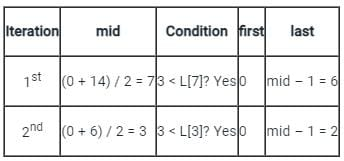Therefore, the value of the variable last is 2 after the first two iterations.
Important points:

• The binary search method works on sorted arrays only.
• It checks the middle element with the search element. If it matches, the index is returned.
• Otherwise, if the search element is lesser than the middle element then the algorithm repeats the process in the left half of the array. Else, it searches in the right half of the array.
Searching Algorithms - Question 5

Example 1:
Consider array has 4 elements and a searching element 16.
A= {10, 16, 22, 25}
The number of iterations are required to search an element by using a binary search= T1

Example 2:
Consider array has 4 elements and a searching element 22.
A= {10, 16, 22, 25}
The number of iterations are required to search an element by using a binary search= T2
Note: Searching is successful.

Q.Which of the following statement are true?

Detailed Solution for Searching Algorithms - Question 5

The correct answer is option 2.
Concept:
Binary Search:
A Binary Search is a sorting method used to find a specific member in a sorted array. Because binary search works only on sorted arrays, an array must be sorted before using binary search on it. As the number of iterations in the binary search reduces, it is a superior searching approach to the liner search technique.
Algorithm:
int binarySearch(int a[], int beg, int end, int K)
{
int mid;
if(end >= beg)
{   mid = (beg + end)/2;
if(a[mid] == K)
return mid+1;
else if(a[mid] < K)
return binarySearch(a, mid+1, end, K);
else
return binarySearch(a, beg, mid-1, K);
}
return -1;
}
Example 1:
The given data,
A= {10, 16, 22, 25}
Searching element= K= 16
beg= 0
end = 3
mid = 3/2 = 1
if K with A[mid] and search is successful.
Hence the number iterations are =1.
Example 2:
The given data,
A= {10, 16, 22, 25}
Searching element= K= 22
beg= 0
end = 3
mid = 3/2 = 1.
Compare 22 with A is not found and K is greater than A[mid]. So  call binarySearch(a, 1+1, 3, 2);
beg= 2
end = 3
mid = 5/2 = 2.
Compare 22 with A is found.
Hence the number of iterations is = 2
Hence the correct answer is T1 < T2.

Searching Algorithms - Question 6

Example 1:
Consider array has 4 elements and a searching element 16.
A= {10, 16, 22, 25}
The number of iterations are required to search an element by using a binary search= T1

Example 2:
Consider array has 4 elements and a searching element 22.
A= {10, 16, 22, 25}
The number of iterations are required to search an element by using a binary search= T2

Note: Searching is successful.
Which of the following statement are true?

Detailed Solution for Searching Algorithms - Question 6

The correct answer is option b.
Concept:

Binary Search:
A Binary Search is a sorting method used to find a specific member in a sorted array. Because binary search works only on sorted arrays, an array must be sorted before using binary search on it. As the number of iterations in the binary search reduces, it is a superior searching approach to the liner search technique.
Algorithm:
int binarySearch(int a[], int beg, int end, int K)
{
int mid;
if(end >= beg)
{   mid = (beg + end)/2;
if(a[mid] == K)
return mid+1;
else if(a[mid] < K)
return binarySearch(a, mid+1, end, K);
else
return binarySearch(a, beg, mid-1, K);
}
return -1;
}
Example 1:
The given data,
A= {10, 16, 22, 25}
Searching element= K= 16
beg= 0
end = 3
mid = 3/2 = 1
if K with A[mid] and search is successful.
Hence the number iterations are =1.
Example 2:
The given data,
A= {10, 16, 22, 25}
Searching element= K= 22
beg= 0
end = 3
mid = 3/2 = 1.
Compare 22 with A is not found and K is greater than A[mid]. So  call binarySearch(a, 1+1, 3, 2);
beg= 2
end = 3
mid = 5/2 = 2.
Compare 22 with A is found.
Hence the number of iterations is = 2
Hence the correct answer is T1 < T2.

Searching Algorithms - Question 7

When two elements map to the same slot in the hash table, it is called ____________?

Detailed Solution for Searching Algorithms - Question 7

The correct answer is option c.
Concept:
Collision:

A collision occurs when more than one value is to be hashed by a particular hash function hash to the same slot in the table or data structure (hash table) being generated by the hash function. (or)
When two elements map to the same slot in the hash table, it is called a collision.
Example:
list of numbers (34, 16, 2, 93, 80, 77, 51) and has a table size is 10.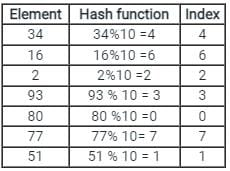The hash table is,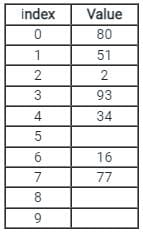The remaining index stores the null values.
Hence the correct answer is Collision.

Searching Algorithms - Question 8

Consider a hash table of size 7, with hash function H (k) = k % 7, and pseudo random i = (i + 5) % 7. We want to insert the following keys one by one from left to right.
15, 11, 25, 16, 9, 8, 12
What will be the position of the key 25, if we use random probing?

Detailed Solution for Searching Algorithms - Question 8

Since we are using random probing:
Insert 15:

(15)%7 = 1

Insert 11:
(11)%7 = 4

Insert 25:
(25)%7 = 4 // collision:
i = 4
i = (i + 5) % 7    // using random function
i = (4 + 5)%7 = 2
Hence 25 position is 2nd

Searching Algorithms - Question 9

What is the worst-case and average-case time complexity of the Binary search?

Detailed Solution for Searching Algorithms - Question 9

Binary search algorithm:

Binary search algorithm is used to find an element in an already sorted array.

STEPS 1:
It finds the middle element of the array and compare the element with the element to be searched, if it matches then return true.

STEPS 2:
If not, then divide the array into two halves in which if element to be search is less than middle element then search takes place in left part otherwise search in right half.

STEP 3:
Repeat this process, until you get the element.

Explanation:
For worst case 52
Worst Case: Search 50 in below give array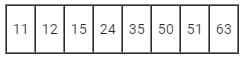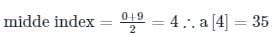50 > 32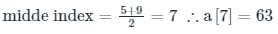50 < 63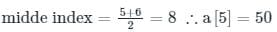matched
T(n) = O(log n)
Also, for average case:
T(n) = O(log n)

Searching Algorithms - Question 10

Consider a hash table with 100 slots. Collisions are resolved using chaining. Assuming simple uniform hashing, what is the probability that the first 3 slots are unfilled after the first 3 insertions?

Detailed Solution for Searching Algorithms - Question 10

Chaining:

• Each slot may contain a chain of elements but in linear probing, we can insert only one element in every slot.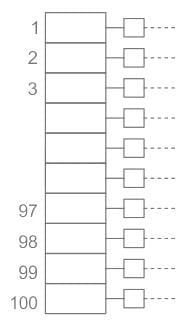• In order to insert element 1, we have 4 to 100 = 97 slots out of 100 slots
• In order to insert second element, we have 4 to 100 = 97 slots out of 100 slots
• In order to insert third element, we have 4 to 100 = 97 slots out of 100 slots
• Required probability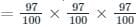Hence option a is the correct option.

## GATE Computer Science Engineering(CSE) 2023 Mock Test Series

149 docs|215 tests
 Use Code STAYHOME200 and get INR 200 additional OFF Use Coupon Code